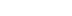/

Pool Hall Lessons: Fast, Accurate Collision Detection Between Circles or Spheres

This feature explains how to detect collisions between two spheres and determine what they'll do after they collide. This is useful not only for games like pool where accurate collision of spheres is key, but also in games where characters and other mobile objects are bounded by spheres, these can be used to quickly determine if they have bumped into each other.

Isn't it wonderful to move through the virtual world in a 3D game? The way you can walk into walls and slide along them as you freely turn -- withoug getting your rail gun stuck between you and the wall? The way you and your opponent can run at each other, swords drawn, actually stop when you reach each other, and can then back away without worrying about your shield getting caught in his chain mail? The way you can circle strafe your opponent over uneven ground without ever having to worry about tripping? In the real world you would have to worry about this kind of stuff…

As developers we can't afford to do collision detection between every polygon of a character and their environment on even the best current hardware; if we did, not only would our games be painfully slow, but we'd also run into problems like those described above. Usually a decent spatial partitioning system and the use of spheres to bound complex, mobile objects let us perform collision detection routines that are very fast and only involve the minimum number of polygons. Not only is it faster, but it helps avoid entangling both player characters and non-player characters in the environment and each other.

Tim Schroeders's article "Collision Detection Using Ray Casting", inthe August 2001 issue of Game Developer magazine focused on detecting collisions between spheres and polygons. This article compliments the information presented there by explaining how to detect collisions between two spheres and determine what they'll do after they collide. This is useful not only for games like pool where accurate collision of spheres is key, but also in games where characters and other mobile objects are bounded by spheres, these can be used to quickly determine if they have bumped into each other.

To make it easier to explain, all the examples will be first in 2D. A later section of this article will explain how to apply the same algorithms to 3D. For the purposes of this article, a circle will be represented by the point at its center and its radius.

Rack 'em! : Proximity Detection between two stationary circles

Are two stationary circles A and B currently touching? The answer, as I'm sure you already know, is very simple. Two circles are in contact with each other if and only if the distance between their centers is less than or equal to the sum of their radii. So, find the distance between the centers of the two circles using the equation: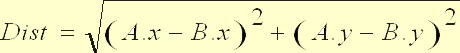Then add the radii of the two circles together. If the sum of the radii is greater than or equal to Dist, then the circles are touching. Since multiplications are less computationally expensive than square roots, you should speed up this code by not performing the square root when calculating the distance, and instead square the sum of the radii. The code below shows a sample implementation using this shortcut.

double deltaXSquared = A.x - B.x; // calc. delta X
deltaXSquared *= deltaXSquared; // square delta X

double deltaYSquared = A.y - B.y; // calc. delta Y
deltaYSquared *= deltaYSquared; // square delta Y

// Calculate the sum of the radii, then square it

// A and B are touching
}

Your Break: Collision between a moving circle and a stationary circle

For this problem you are given a circle A that is moving through a virtual world. Over a finite period of time, which we'll call t, A moves a certain distance in a certain direction. We'll represent this movement by a vector V. Also in this virtual world is a circle B that is not moving. The problem is to figure out if A comes into contact with B while it is moving along V, and if it does, by what amount do we have to shorten V so that A comes to rest just at the point of contact with B? An illustration of the problem is shown in figure 2.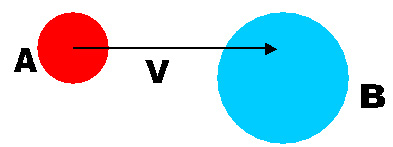Figure 2: Illustration of the Dynamic-Static Collision Problem

Leveraging Stationary Collision

The first, and most obvious solution to this would be to use the stationary collision method described above on the changing destination location of circle A. In other words, move circle A to the end of the movement vector and then test it's new position for collisions. If A is in contact with another circle in its new location, you have two choices. You could do a recursive back off, where you shorten the movement vector and try again until A and B are not touching. Or you could simply not move A. These methods have several problems.

If you went with the recursive back off solution, you could potentially eat up a lot of CPU time trying to make the collision appear accurate. Or, you could set a limit on the number or retries, reducing the computations to an acceptable load but then leaving you potentially with the same problems with the other option…

You could not move the circle at all. It's really cheap to do, but nothing would ever seem to really touch. In the game, circle A would move towards a static circle B over several frames until it's new position intersected with B. Then it would appear to just stop dead some amount before it would have hit B, as if the static circle B had some kind of invisible force field built around it. Assuming you are doing collision detection based on frame rate, the effects would be more noticeable as the frame rate drops.

Finally, if A is moving fast, it is possible that A's final destination is on the other side of B, but not touching it. You would have to perform additional checks to make sure A did not pass through any other object.

Clipping the Movement Vector

A better approach would be to use the movement vector V to represent the circle as it moves through the world. Now all the collision detection is simplified down to a vector tested against a static circle. This approach does one test to see if V touches B. If it does not collide then you are done testing.

This solution has problems as well. Consider the situation shown in figure 3. The movement vector V from the center of A does not come into contact with anything. However, it only checks the path traveled by the center of A for collision, and the bottom or top could still collide with B even if the middle does not.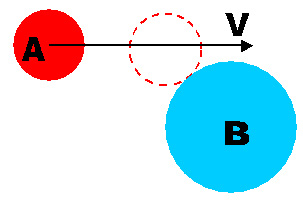Figure 3

A possible solution to this problem is to test against two more vectors coming from the edges of the moving circle parallel to the movement vector V. While this may fix the problem described above, we can see in figure 4 that if we adjust the movement vector V to be the length of the clipped second vector, the circles will still overlap. Also, if the moving circle is larger than the static one, the static one might fit between the top and center vectors, or between the center and bottom vectors, so the collision would not be detected at all. The moving circle would appear to go right over the smaller static one. Obviously this is not the correct answer for collision detection.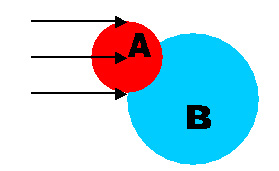Figure 4

An Algorithm That Works

The first step to the right solution is to quickly determine that there can be no collision and avoid doing any more tests. So first we figure out if A is going far enough that it could conceivably hit B. That means that the movement vector must be at least as long as the shortest distance between the circles, which would be a straight line passing through their centers. So the movement vector must be at least the distance between the centers of the circles minus the radius of each. If it is not, then there is no way that the circles will collide. Note that this test does not take direction into account! Thus it does not tell us that A and B will collide; it tells us that they won't. See Figure 5 for an illustration.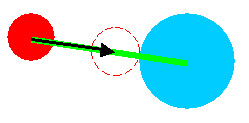Figure 5: The shortest length the movement vector can be for A and B to collide

Our next early escape test is to determine if A is actually moving towards B. If it's not, then obviously you don't have to worry about them colliding. To do this, we take advantage of our friend, the Dot Product. First, find C, the vector from the center of A to the center of B. Remember that a point minus a point is a vector, so C = B - A. Now get the dot product of C and the movement vector, V: if the result is less than or equal to 0, then A is not moving towards B, and no more testing needs to be done.

One more escape test: If the closest that A ever gets to B is more than the sum of their radii, then they do not hit. Dot product to the rescue again! If theta is the angle between any two vectors P and Q, then the dot product between P and Q is equivalent to: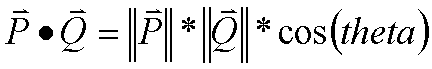In other words, the dot product of two vectors P and Q is equal to the cosine of the angle between them times the length of P and the length of Q.

Also recall that the cosine of an angle is equal to the side of a right triangle adjacent to that angle, divided by the hypotenuse of that same triangle. Therefore, the dot product of a vector P and a normalized (ie: has a length of 1) vector Q is equal to the length of P times the cosine between the two vectors. Which, in turn, is equal to the length of the vector P in the direction of the normalized vector Q. This is shown in Figure 6.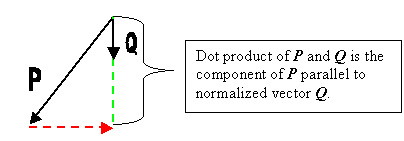Figure 6

With this in mind, lets go back to the problem at hand. We have our movement vector V, and our vector from the center of circle A to the center of circle B, called vector C. We want to find the point on V that is closest to the center of B. Intuitively, if we were to draw a line from this point to the center of B, it would be at right angles to V. Therefore, we can use the dot product as described above to find the distance from the center of A to that point. Compute the normalized V (call it N) and then take the dot product of N and C. The result will be the floating point number D, the distance between the center of A and the closest point on V to B. See Figure 7 for a visual reference.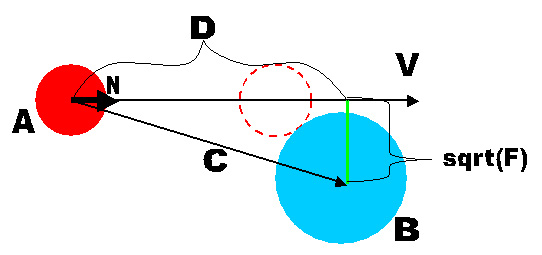Figure 7: Calculating the closest point on V to B

The length of C and D are the lengths of two sides of a right triangle. Thus, we can use the Pythagorean Theorem (a^2 + b^2 = c^2) to find the length of the third side, represented in green in figure 7. Square the length of C, and subtract the square of D from it, and call the result F.
Now, to be accurate, the square root of F is the length from the center of B to the closest point to B on V. However, performing square roots take a lot of processor time. So we will perform our early escape test without taking the square root of F. What we need to know is, as stated before, do A and B touch when A is at the closest point to B along V? In other words, is sqrt(F) <= A.radius + B.radius? But rather than take the square root of F, check F <= (A.radius + B.radius)^2. If this comparison is false, then there is no collision and you can escape the routine.

At this point we've exhausted our early escape tests, and there is still a chance that the two circles will collide.

Figure 8 gives a visual explanation of the steps about to be described. The distance circle A can move before colliding with B is right up until it is just touching the edge of circle B. At that point, the distance between the centers of the circles is equal to the sum of the radii. Since we already know the shortest distance from V to the center of B, aka sqrt(F), we have the lengths of two sides of a right triangle. The third side is equal to D - distance A can travel before it hits B. So again we can use the Pythagorean theorem, and find the length T = (A.radius + B.radius)^2 - F. The distance A has to move along V to come into contact with B is D - sqrt(T).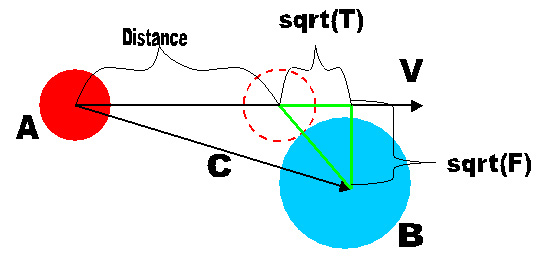Figure 8: Finding the farthest that A can go

One final check is needed. Remember when we tested the shortest distance between A and B, but it did not take into account direction? Here's where that can come back and bite us. Consider the situation illustrated in figure 9. Both arrows are the same length, but in slightly different directions. This shows that yes, the movement vector is long enough to bring the two circles close enough to touch, but the direction is such that they won't. So at this point in the algorithm we need to do a reality check: if Distance is greater than the length of V, then there is no collision.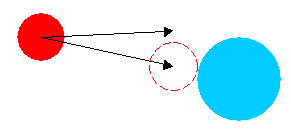Figure 9

If the final test is passed, we can normalize V and then multiply it by D - sqrt(T). Now Circle A will move until it is just touching circle B. The Java code implementing this algorithm is listed below.

// Early Escape test: if the length of the movevec is less
// than distance between the centers of these circles minus
// their radii, there's no way they can hit.
double dist = B.center.distance(A.center);
if(movevec.Magnitude() < dist){
return false;
}

// Normalize the movevec
Vector N = movevec.copy();
N.normalize();

// Find C, the vector from the center of the moving
// circle A to the center of B
Vector C = B.center.minus(A.center);

// D = N . C = ||C|| * cos(angle between N and C)
double D = N.dot(C);

// Another early escape: Make sure that A is moving
// towards B! If the dot product between the movevec and
// B.center - A.center is less that or equal to 0,
// A isn't isn't moving towards B
if(D <= 0){
return false;
}

// Find the length of the vector C
double lengthC = C.Magnitude();

double F = (lengthC * lengthC) - (D * D);

// Escape test: if the closest that A will get to B
// is more than the sum of their radii, there's no
// way they are going collide
return false;
}

// We now have F and sumRadii, two sides of a right triangle.
// Use these to find the third side, sqrt(T)
double T = sumRadiiSquared - F;

// If there is no such right triangle with sides length of
// sumRadii and sqrt(f), T will probably be less than 0.
// Better to check now than perform a square root of a
// negative number.
if(T < 0){
return false;
}

// Therefore the distance the circle has to travel along
// movevec is D - sqrt(T)
double distance = D - sqrt(T);

// Get the magnitude of the movement vector
double mag = movevec.Magnitude();

// Finally, make sure that the distance A has to move
// to touch B is not greater than the magnitude of the
// movement vector.
if(mag < distance){
return false;
}

// Set the length of the movevec so that the circles will just
// touch
movevec.normalize();
movevec.times(distance);

return true;

Bank Shot: Collision between two moving circles

This problem seems even more complicated than the previous one. Given two moving circles, determine whether or not they collide. Looking at the problem in figure 10, we can see that just because their paths cross does not mean that the circles will come into contact. One may have moved out of the way in time. It also shows that, just because their paths do not cross does not mean that they don't collide.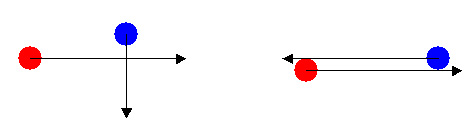Figure 10: the Dynamic-Dynamic collision problem

Thankfully, the solution to a very hard problem could not be simpler. What we are really interested in is not their movement vectors, but their movement relative to each other. If we translate circle A's movement such that B can be considered stationary, we can use the Dynamic-Static solution described above!

First of all, subtract the movement vector of one circle from another (or, you can think of it as adding the reverse of one vector to another). Then perform the dynamic-static collision algorithm on the circles and the new vector. If they collide, divide the length of the shortened vector by the length of the one you originally passed into the function. The result should be a floating-point number between 0 and 1. This represents when over the course of their movement the circles collided. Multiply the original movement vectors by this number, and the result is shortened movement vectors that take the circles up to the point where they touch for the first time.

Jumping the Cue ball: Applying these algorithms to 3D

Detecting proximity between two stationary spheres
The application of this algorithm to 3D environments couldn't be easier. Circles in 2D and spheres in 3D are both defined the same way mathematically: Given a center point, the boundaries of both circles and spheres are defined as all points that are a given distance (the radius) from the center point. So spheres can be mathematically specified in the same way as circles with a center point and a radius. There is one caveat for the 3D representation. The center point in 3D uses a third coordinate, z. So the distance between the centers of the two spheres can be found by: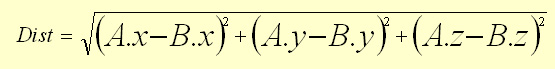If that distance is less than the sum of the radii of the two spheres, then they are in contact with each other.

Collision of a moving sphere with a stationary sphere

The Dynamic-Static collision algorithm works in 3D because the 3D scenario can be reduced to 2D. If you look at our solution for this problem in 2D, you'll notice that it is based around two vectors (V and C) and a common point (the center of A). These two vectors define the orientation of the plane in 3D, and the point provides a reference to where in space that plane lies. Figures 11 and 12 show two spheres, the red one in motion and the blue one at rest. These figures also show the movement vector of the red one and the vector between the centers of the spheres. Notice the 2D plane that is passing through the objects in the scene in both figures. This plane cuts right down the middle of the two spheres and along the two vectors, clearly showing that these vectors are coplanar. Also notice that the plane cuts the spheres perfectly in half, so that the areas of contact between the spheres and the plane are circles with the same center and radius as the spheres. All of the information needed to use the dynamic-static algorithm we described above is projected into a 2D space on the light blue plane.

There is a special case to be considered, although its resolution is trivial. If the vectors V and C lie along the same line, then there are an infinite number of planes in which the problem can be solved. This case should be checked for. Of course, if V and C are co-linear, that means that sphere A is moving directly towards sphere B, and the question of whether they collide reduces to only our first early escape test. Namely, "does A move far enough to hit B?", or in other words, "Is V greater than (C - sumRadii)?"

There are no changes needed to the code above in order for it to work in the 3D case. The changes that need to be done are in the classes that are assumed by the above code. For example, instead of the Vector class having only x and y member variables, it should be changed to include a third member variable, z.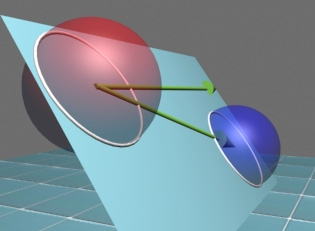Figure 11: Dynamic-Static Collision in 3D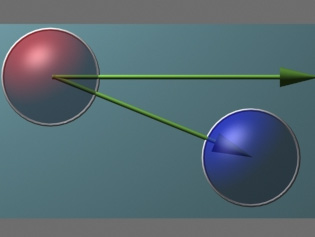Figure 12: The Dynamic-Static Collision, projected in 2D

Collision between two moving spheres

Again, by translation of the problem to one sphere's frame of reference, this problem is reduced to the Dynamic-Static 3D problem, which in turn scales down to the 2D case, as described above. Figure 13 shows two spheres, each with it's own movement vector, shown in green. The orange vector is opposite of B's movement vector, and the yellow movement vector from A (the red ball) is the movement of A as observed from B's point of reference. The 2D plane containing the yellow vector also contains the center of sphere B, and so it can be used to solve the problem.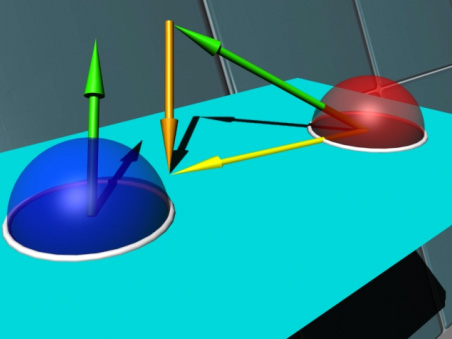Figure 13: Collision of two moving spheres in 3D, and how it can be reduced to one moving circle in 2D

Now that you have determined that your circles collide, you want to have them bounce off of each other in a realistic manner, taking into account their relative mass and speed. To solve this, we are going to rely on some simple laws of physics, specifically the conservation of momentum and the conservation of energy.

Look at figure 13 to get an idea of the problem and the variables that we are going to use. The red circle is circle1, the blue one circle2. They each have a movement vector, movevec1 and movevec2, and a mass, m1 and m2.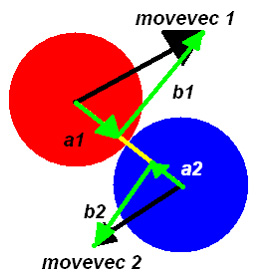Figure 14: Bounce

Conservation of Momentum states that the total momentum of the system before the collision is equal to the total momentum in the system after the collision. If we represent the momentum of a circle to be P = M * V, where M is the circles mass and V is its movement vector, then we can derive the equation: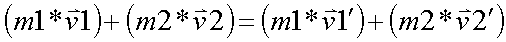where v1'and v2' are the movement vectors of circle 1 and 2 respectively after the collision. Since the second circle gains any momentum lost by the first, we can represent the difference between the momentums of the balls before and after by the same vector, deltaP.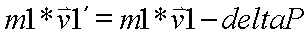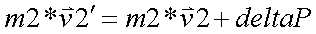Now here is where the difference between reality and simulation comes into play. If these two spheres were the rubber balls we all used in gym class in high school, when they hit they would deform. This deformation would increase the area where the balls are touching, and some of the energy would be lost in that deformation. Other amounts of it would be lost in spin. But in this simulation, we are assuming the balls to be rigid, frictionless, perfect spheres. A common real-world example of this type might be the steel balls hanging from a frame that collide with each other to demonstrate action-reaction; because they are so rigid, very little of their momentum is lost when they collide, and so when you set one ball swinging it takes some time for them all to stop.

So in our simulation of perfect rigid spheres, the only transference of momentum can occur along the single point of contact, as illustrated in figure 13. Therefore, we can break deltaP into a unit vector N that points down the line of contact, and a scalar P representing the magnitude of deltaP. So, if we apply this to the equations above, we can solve for the new movement vectors of the circles and get: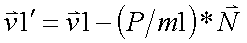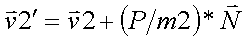So, if we can solve for P, we can calculate the new movement vectors.

Now look back at figure 13, and notice that v1 and v2 can be represented by the sum of two vectors: one that is parallel to the line along which momentum is exchanged, and one that is perpendicular to it. Using this information, we can represent v1, v1', v2, and v2' by: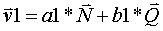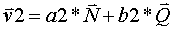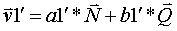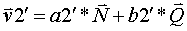Where a1, a2, b1, and b2 are scalars, N is the same N as mentioned before, and Q is the normalized vector perpendicular to the line along which momentum is exchanged and on the same plane as N and the movement vector.

Substituting v1 in equation 1 for the value of v1 in equation 3, and v2 in equation 2 for the value of v2 in equation 3, we get: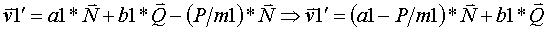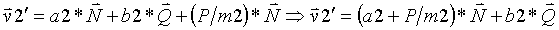And since v1' = a1'*N + b1'*Q and v2' = a2'*N + b2'*Q, we can see that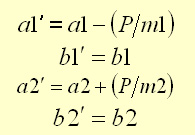Now we can use the Conservation of Energy to solve for P. The equation for kinetic energy is: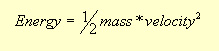Since energy is conserved, the total energy before the collision must equal the total energy after the collision: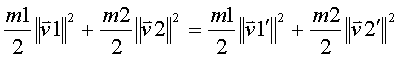Using the movement vector as the hypotenuse of a right triangle, we can substitute: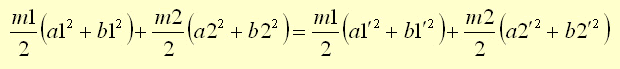Substituting equation 4 for a1', b1', a2' and b2', we get: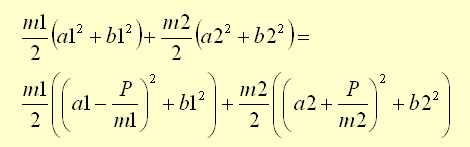Note that the b1 and b2 terms in equation 7 drop out of the equation. With an equation in terms of m1, m2, a1, a2, and P, we have an equation with variables that are either given or can be calculated from what was given, except for P. So if we solve for P, we will be able to plug in the known variables, derive P, and then use P to calculate the new movement vectors. Equation 8 shows equation 7 after solving for P.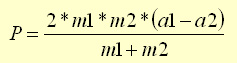So, plugging this into Equations 1 & 2: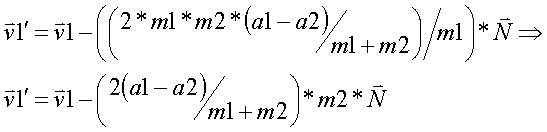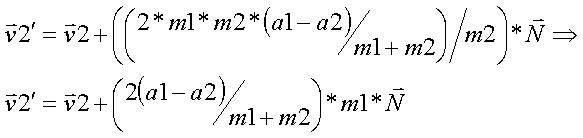Notice that for both v1' and v2' the term in the () brackets is the same, so you only need to calculate that once. With that done, you can calculate v1'and v2'. Now that we've done the math for this, the code to implement the results is very short and quick. The variable optimizedP in the code refers to the term in brackets above.

// First, find the normalized vector n from the center of
// circle1 to the center of circle2
Vector n = circle1.center - circle2.center;
n.normalize();

// Find the length of the component of each of the movement
// vectors along n.
// a1 = v1 . n
// a2 = v2 . n
float a1 = v1.dot(n);
float a2 = v2.dot(n);

// Using the optimized version,
// optimizedP =  2(a1 - a2)
//              -----------
//                m1 + m2
float optimizedP = (2.0 * (a1 - a2)) / (circle1.mass + circle2.mass);

// Calculate v1', the new movement vector of circle1
// v1' = v1 - optimizedP * m2 * n
Vector v1' = v1 - optimizedP * circle2.mass * n;

// Calculate v1', the new movement vector of circle1
// v2' = v2 + optimizedP * m1 * n
Vector v2' = v2 + optimizedP * circle1.mass * n;

circle1.setMovementVector(v1');
circle2.setMovementVector(v2');

8 Ball, Corner Pocket

These techniques will allow you use spheres with a higher degree of accuracy than is probably necessary if your spheres are all bounding spheres. Precise collisions between spheres become important when simulating things likes pool balls or marbles, or potentially rocks in a landslide. But for spheres bounding characters, for example, you might not care what angle two colliding characters would bounce away at. However, parts of these methods are fast enough to be useful if only to determine that a collision was avoided. But who knows; using a pumped-up space marine as a cue ball just might be humorous enough to do…

Special thanks to Dave Baum for his help with collision response.

______________________________________________________

Latest Jobs

Pocketwatch Games

Remote
01.05.22
Senior Gameplay Engineer

Disbelief

Cambridge, Massachusetts
01.07.22
Jr. Programmer

Gameloft Australia

Brisbane, Australia
01.06.21
Creative Director

Sucker Punch Productions

Bellevue, Washington
01.06.22
Senior Systems Designer
More Jobs